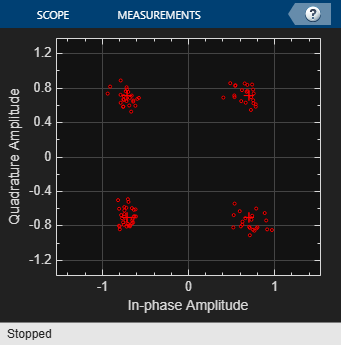# QPSK Modulator Baseband

Modulate using quadrature phase shift keying method

## Library

PM in Digital Baseband sublibrary of Modulation

•## Description

The QPSK Modulator Baseband block modulates using the quadrature phase shift keying method. The output is a baseband representation of the modulated signal.

### Integer-Valued Signals and Binary-Valued Signals

If you set the Input type parameter to `Integer`, then valid input values are 0, 1, 2, and 3. When you set Constellation ordering to `Binary` for input m the output symbol is

exp(jθ + jπm/2)

where θ represents the Phase offset parameter (see the following figure for Gray constellation ordering). In this case, the block accepts a scalar or column vector signal.

If you set the Input type parameter to `Bit`, then the input contains pairs of binary values. For this configuration, the block accepts column vectors with even lengths. When you set the Phase offset parameter to $\frac{\Pi }{4}$, then the block uses one of the signal constellations in the following figure, depending on whether you set the Constellation ordering parameter to `Binary` or `Gray`.In the previous figure, the most significant bit (i.e. the left-most bit), is the first bit input to the block. For additional information about Gray mapping, see the M-PSK Modulator Baseband help page.

### Constellation Visualization

The QPSK Modulator Baseband block provides the capability to visualize a signal constellation from the block mask. This Constellation Visualization feature allows you to visualize a signal constellation for specific block parameters. For more information, see the Constellation Visualization section of the Communications Toolbox™ User's Guide.

## Dialog BoxThe phase of the zeroth point of the signal constellation.

Constellation ordering

Determines how the block maps each pair of input bits or input integers to constellation symbols.

Input type

Indicates whether the input consists of integers or pairs of bits.

Output data type

The output data type can be set to `double`, `single`, `Fixed-point`, `User-defined`, or ```Inherit via back propagation```.

Setting this parameter to `Fixed-point` or `User-defined` enables fields in which you can further specify details. Setting this parameter to `Inherit via back propagation`, sets the output data type and scaling to match the following block.

Output word length

Specify the word length, in bits, of the fixed-point output data type. This parameter is only visible when you select `Fixed-point` for the Output data type parameter.

Set output fraction length to

Specify the scaling of the fixed-point output by either of the following methods:

• Choose `Best precision` to have the output scaling automatically set such that the output signal has the best possible precision.

• Choose `User-defined` to specify the output scaling in the Output fraction length parameter.

This parameter is only visible when you select `Fixed-point` for the Output data type parameter or when you select `User-defined` and the specified output data type is a fixed-point data type.

User-defined data type

Specify any signed built-in or signed fixed-point data type. You can specify fixed-point data types using the `sfix`, `sint`, `sfrac`, and `fixdt` functions from Fixed-Point Designer™. This parameter is only visible when you select `User-defined` for the Output data type parameter.

Output fraction length

For fixed-point output data types, specify the number of fractional bits or bits to the right of the binary point. This parameter is only visible when you select `Fixed-point` or `User-defined` for the Output data type parameter and `User-defined` for the Set output fraction length to parameter.

## Examples

expand all

Open the QPSK model. The model generates QPSK data, applies white noise, and displays the resulting constellation diagram.Run the model.Change the Eb/No of the AWGN Channel block from 15 dB to 10 dB.The noise level increases as shown by the greater distance between the samples.

## Supported Data Types

PortSupported Data Types

Input

• Double-precision floating point

• Single-precision floating point

• Boolean when Input type is `Bit`

• 8-, 16-, and 32-bit signed integers

• 8-, 16-, and 32-bit unsigned integers

• ufix(1) when Input type is `Bit`

• ufix(2) when Input type is `Integer`

Output

• Double-precision floating point

• Single-precision floating point

• Signed fixed point

## Pair Block

QPSK Demodulator Baseband